# Angle facts for kids

Kids Encyclopedia Facts

When two straight lines come together, they make an angle. The two lines are called the sides of the angle, and they meet at a point. A flat surface (called a plane) also forms an angle when it meets another.

To represent an angle, Greek letters such as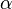$\alpha$ (alpha),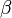$\beta$ (beta),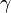$\gamma$ (gamma) and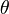$\theta$ (theta) are sometimes used. An angle indicates the space between its sides, or the amount of rotation needed to make one side coincide the other.

To measure the size of an angle, we use units called degrees. A degree is a standard unit and we use the symbol ° after a number to show that it is a number of degrees. We can use a decimal number or a fraction for part of a degree, but a degree can also be divided into 60 minutes (1° = 60'), and a minute can be divided into 60 seconds (1' = 60"). So 22.5°, 2212° and 22° 30' are all the same angle.

In mathematics, angles can also be (and often are) measured in radians instead of degrees, by using the conversion factor$2\pi\mbox{ rad} = 360^\circ$ (for example,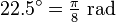$22.5^\circ = \tfrac{\pi}{8}\mbox{ rad}$). Yet another unit of angle is gradian, with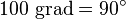$100 \text{ grad} = 90^{\circ}$.

Angles are studied in geometry, where an angle where edges meet is often called a vertex. For example, the three sides of a triangle are its edges and two of the edges meet at each vertex. Similarly, two of the six sides (or faces) of a cube meet at each of its twelve edges, and three edges meet at each of its eight corners (or vertices, which is the plural version of vertex).

## Types of angles

An acute angle is an angle less than 90° (but more than 0°). A right angle is an angle equal to 90°. An obtuse angle is an angle greater than 90° but less than 180°. A straight angle (or straight line) is an angle equal to 180°. A reflex angle is an angle greater than 180° (but less than 360°).

Supplementary angles are two angles with the sum equal to 180°, and complementary angles are two angles with the sum equal to one right angle (90°). On the other hand, two angles that sum to one full circle (360°) are sometimes called explementary angles, or conjugate angles.

People usually use a protractor to measure and draw angles. Sometimes, people use a 360° protractor to measure angles.

## Images for kidsAngle Facts for Kids. Kiddle Encyclopedia.